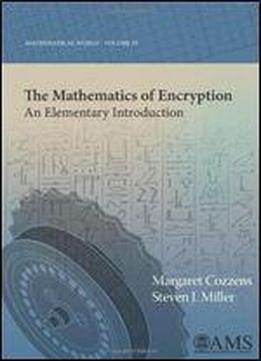# The Mathematics Of Encryption: An Elementary Introduction by Margaret Cozzens / 2013 / English / PDF

How quickly can you compute the remainder when dividing by 120143? Why would you even want to compute this? And what does this have to do with cryptography? Modern cryptography lies at the intersection of mathematics and computer sciences, involving number theory, algebra, computational complexity, fast algorithms, and even quantum mechanics. Many people think of codes in terms of spies, but in the information age, highly mathematical codes are used every day by almost everyone, whether at the bank ATM, at the grocery checkout, or at the keyboard when you access your email or purchase products online. This book provides a historical and mathematical tour of cryptography, from classical ciphers to quantum cryptography. The authors introduce just enough mathematics to explore modern encryption methods, with nothing more than basic algebra and some elementary number theory being necessary. Complete expositions are given of the classical ciphers and the attacks on them, along with a detailed description of the famous Enigma system. The public-key system RSA is described, including a complete mathematical proof that it works. Numerous related topics are covered, such as efficiencies of algorithms, detecting and correcting errors, primality testing and digital signatures. The topics and exposition are carefully chosen to highlight mathematical thinking and problem solving. Each chapter ends with a collection of problems, ranging from straightforward applications to more challenging problems that introduce advanced topics. Unlike many books in the field, this book is aimed at a general liberal arts student, but without losing mathematical completeness.

views: 716